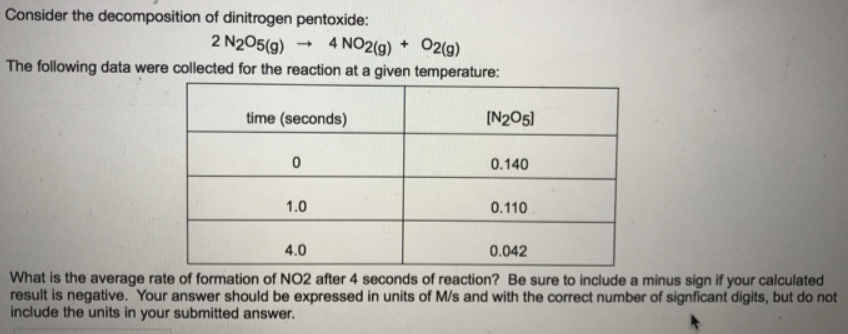# Problem: Consider the decomposition of dinitrogen pentoxide: 2 N2O5 (g) → 4 NO2 (g) + O2 (g) The following data were collected for the reaction at a given temperature: What is the average rate of formation of NO2 after 4 seconds of reaction? Be sure to include a minus sign if your calculated result is negative. Your answer should be expressed in units of M/s and with the correct number of significant digits, but do not include the units in your submitted answer.

###### FREE Expert Solution###### Problem Details

Consider the decomposition of dinitrogen pentoxide:

2 N2O5 (g) → 4 NO2 (g) + O2 (g)

The following data were collected for the reaction at a given temperature:

What is the average rate of formation of NO2 after 4 seconds of reaction? Be sure to include a minus sign if your calculated result is negative. Your answer should be expressed in units of M/s and with the correct number of significant digits, but do not include the units in your submitted answer.